﻿ 氢同位素分离柱的循环冷却数学模型 Mathematical Model of Hydrogen Isotope Separation Column in Circulating Cooling Condition

Nuclear Science and Technology
Vol.05 No.03(2017), Article ID:21513,7 pages
10.12677/NST.2017.53022

Mathematical Model of Hydrogen Isotope Separation Column in Circulating Cooling Condition

Xiangbo li, Shilin Hu, Yi Kang, Hailin Bai

China Institute of Atomic Energy, BeijingReceived: Jul. 10th, 2017; accepted: Jul. 23rd, 2017; published: Jul. 26th, 2017ABSTRACT

Based on the classic Newton’s law of cooling and combined with the mathematical methods such as differential equation, iterative method, least square method and linear fitting analysis, a new mathematical model is established. The mathematical model can be used to analyze the cooling curve of hydrogen isotope separation column which was cooled by both natural ventilation cooling and circulating cooling, predict the required cooling time and guide the selection of cooling conditions.

Keywords:Cooling Mathematical Model, Newton’s Law of Cooling, Iterative Method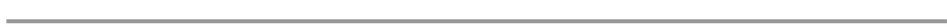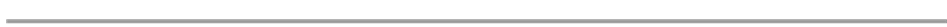Copyright © 2017 by authors and Hans Publishers Inc.

This work is licensed under the Creative Commons Attribution International License (CC BY).

http://creativecommons.org/licenses/by/4.0/1. 引言

2. 实验装置

3. 自然冷却条件下的冷却模型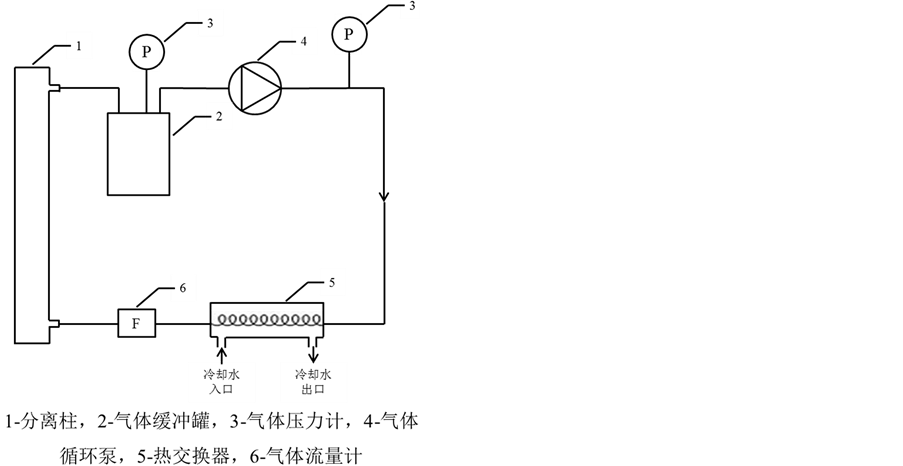Figure 1. Flowchart of circulating cooling simulation experiment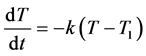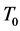为分离柱初始温度。以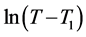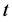作线性拟合，即可求出冷却系数Figure 2. Temperature curve of separating column under natural cooling conditions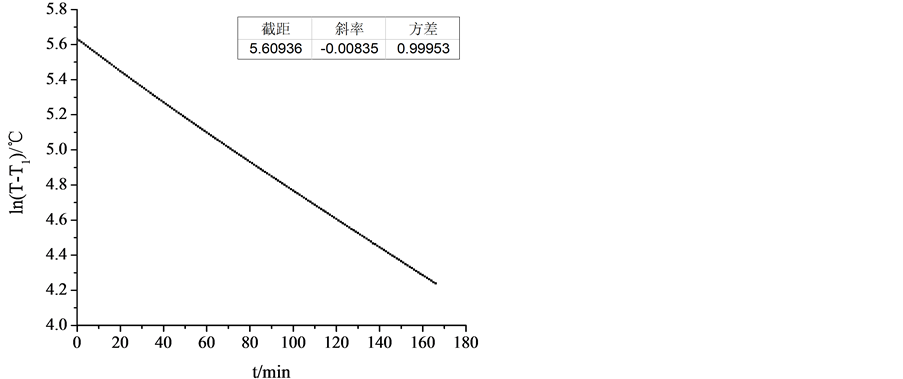Figure 3. Linear fitting results under natural cooling conditions

4. 循环冷却条件作用下的冷却模型

(1) 冷却气温度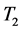= 环境温度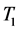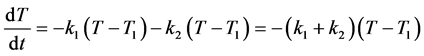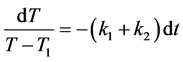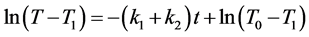作线性拟合，其斜率即为。由此可知，若冷却气温度等于室温，则总的冷却系数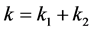，即二者简单加和可得。由于自然冷却系数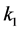可事先由牛顿冷却模型求得，且并不因循环冷却的存在而改变，因此可求得循环冷却系数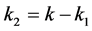(2) 冷却气温度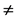环境温度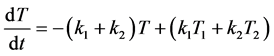作一次线性拟合，其斜率为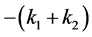，进而求出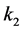。将求出的代入，并以作二次线性拟合，则可得到一个新的斜率值，进而求出一个新的值。将得到的新继续代入公式中拟合，直到代入的值与拟合得到的值相等，最终求得的即为真实值。Figure 4. Temperature curve of separating column under circulating cooling conditions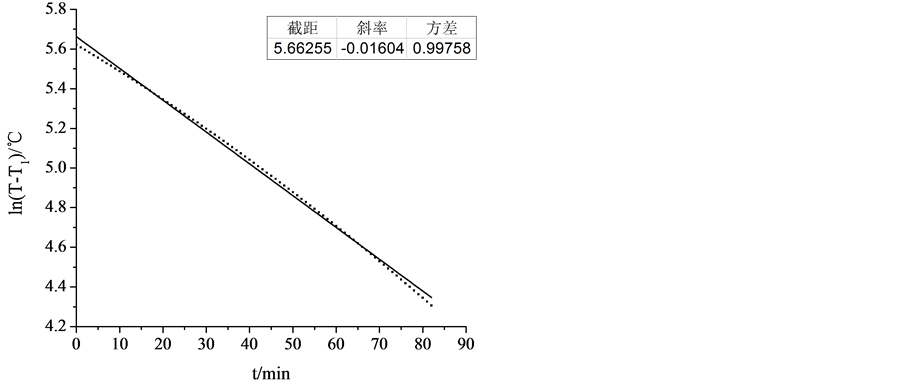Figure 5. First linear fitting results under circulating cooling conditions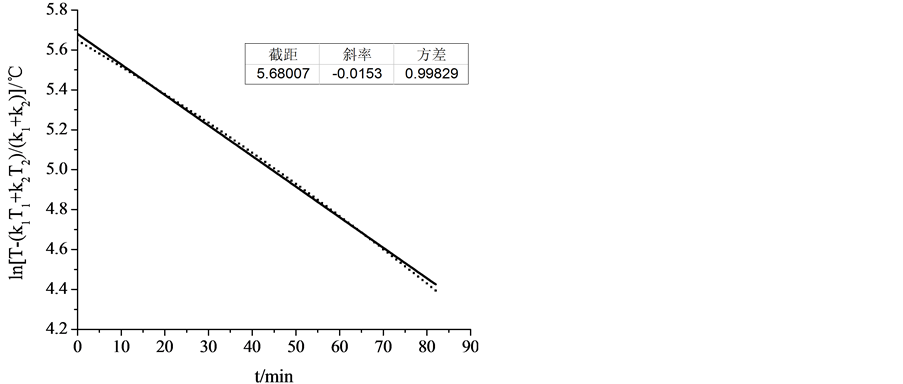Figure 6. Fourth linear fitting results under circulating cooling conditions

5. 总结Mathematical Model of Hydrogen Isotope Separation Column in Circulating Cooling Condition[J]. 核科学与技术, 2017, 05(03): 170-176. http://dx.doi.org/10.12677/NST.2017.53022

1. 1. 蒋国强, 罗德礼, 陆光达, 孙灵霞. 氚和氚的工程技术[M]. 北京: 国防工业出版社, 2007.

2. 2. 彭述明, 王和义. 氚化学与工艺学[M]. 北京: 国防工业出版社, 2015.

3. 3. 霍尔曼J.P., 等. 传热学[M]. 北京: 人民教育出版社, 1979.

4. 4. 过增元. 热流体学[M]. 北京: 清华大学出版社, 1992.

5. 5. 菲赫金哥尔茨. 微积分学教程[M]. 北京: 高等教育出版社, 2006.